# Half Section in Four Terminal Network

## Half Section in Four Terminal Network:

The Half Section in Four Terminal Network can be obtained by splitting symmetrical T and π networks into two identical networks. The half sections obtained by splitting shunt arm of symmetrical T network in two equal impedances and series arm of symmetrical π network in two equal impedances are identical as shown in the Fig. 8.24 (a) and (b).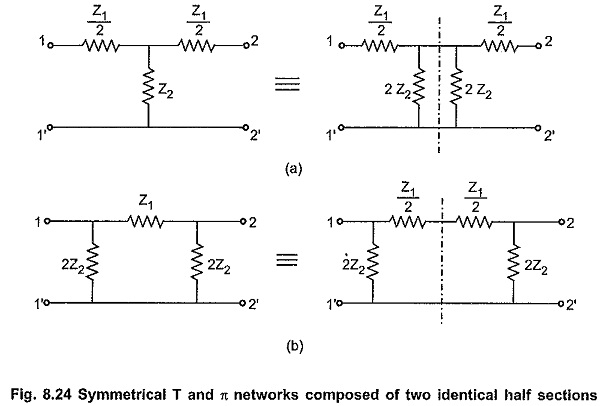Consider a half section as shown in the Fig. 8.24. As half section is of asymmetrical type, we have to analyze this network on the basis of iterative impedances and image impedances separately.

### Iterative Impedances (Z′0):

By the property of asymmetrical network, the iterative impedances at the two ports of the network are of different value and they are represented by Z′01 and Z′02.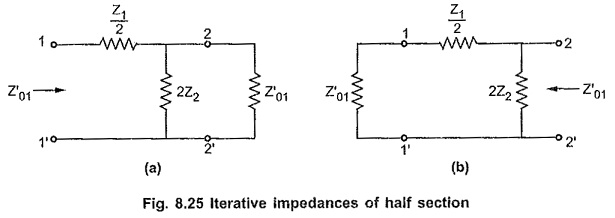Consider Fig. 8.25 (a),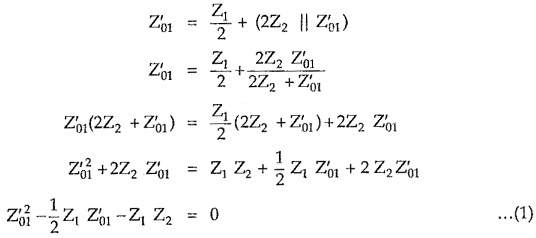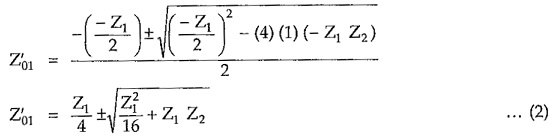Consider Fig. 8.25 (b),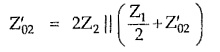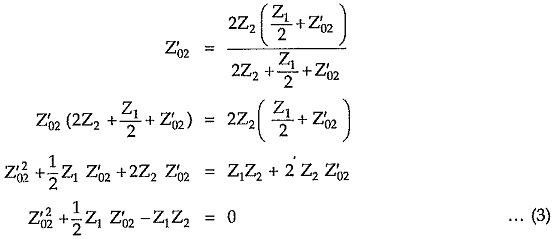Find roots of above equation we can write,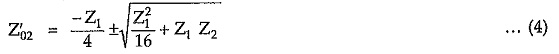### Image Impedances (Zi):

For any asymmetrical network, the image impedance at any port is the geometric mean of open and short circuit impedances at that port.

If Zi1 is the image impedance at port 1, then it is given by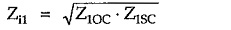Similarly if Zi2 is the image impedance at port-2, then it is given by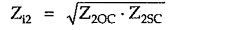Consider a half section without any termination as shown in the Fig. 8.26.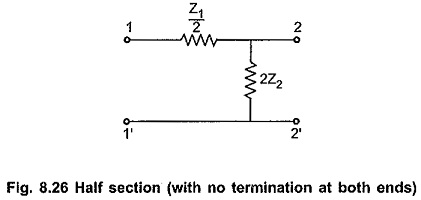The impedances at terminals 1 – 1′ with terminals 2 – 2′ open and short circuited are represented by Z1OC and Z1SC respectively. These impedances are given by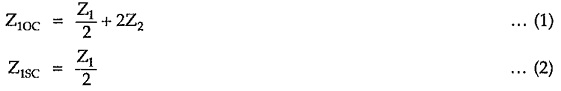Thus, image impedance Zi1 at port 1 is given by,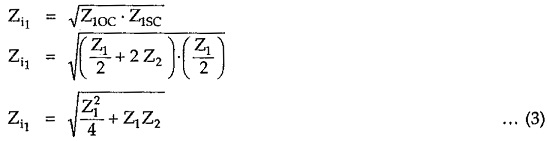The impedances at terminals 2 – 2′ with terminals 1 – 1′ open and short circuited are represented by Z2OC and Z2SC respectively. These impedances are given by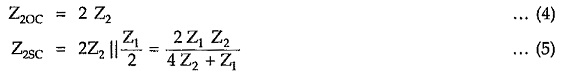Thus, image impedance Zi2 at port 2 is given by,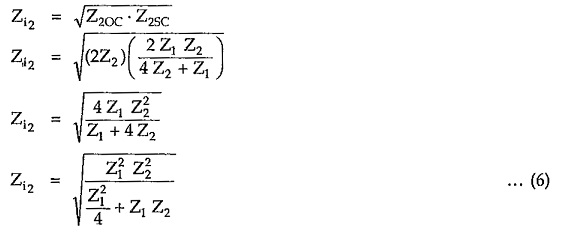From equations (3) and (6) it is clear that the image impedances at port 1 and port 2 are the characteristic impedances of symmetrical T and π networks having total series and shunt arm impedances as Z1 and Z2 respectively. Because of this property of the half section, it can be used to match a T section with a π section if both are having same series and shunt arm impedances.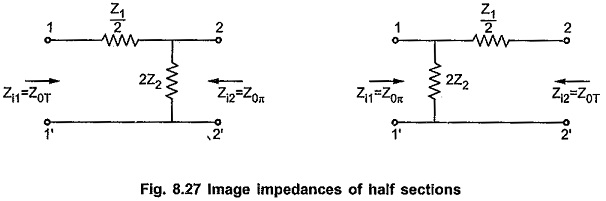Updated: November 9, 2019 — 5:49 pm